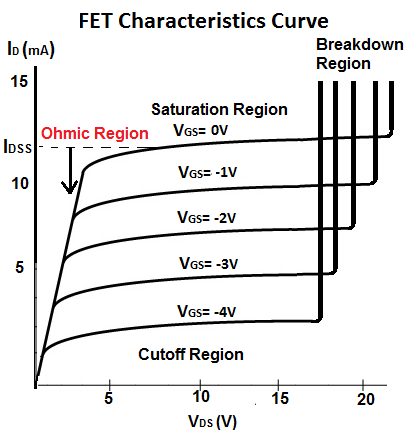﻿ What is the Ohmic Region of a FET Transistor# What is the Ohmic Region of a FET Transistor?The ohmic region of a FET transistor (also called the linear region) is the region where the drain current, ID, has a linear response to changes in the drain-source voltage, VDS, which mimics the linear response that would be obtained from Ohm's Law.

The drain current ID depends on the drain-source voltage VDS. Right at the beginning of the curve, when VDS is small, the drain current ID varies nearly linearly with VDS.

This the region where the JFET is beginning to resist. It acts like a variable resistor.

The Ohmic Region is the only region on a FET Characteristics curve where there is a linear response in current from changes in the voltage.

It is called the ohmic region, or linear region, because the JFET behaves like a voltage-controlled resistor. It mimics ohm's law, where current has a linear response to changes in voltage (I=V/R). In other parts of the characteristics curve, the current behaves nonlinearly, nothing as would occur in ohm's law.